Updating search results...

# 33 Results

View
Selected filters:
• MCCRS.Math.Content.6.G.A.1Only Sharing Permitted
CC BY-NC-ND
Rating
4.5 stars

This lesson unit is intended to help teachers assess how well students are able to visualize two-dimensional cross-sections of representations of three-dimensional objects. In particular, the lesson will help you identify and help students who have difficulties recognizing and drawing two-dimensional cross-sections at different points along a plane of a representation of a three-dimensional object.

Subject:
Geometry
Material Type:
Assessment
Lesson Plan
Provider:
Shell Center for Mathematical Education
Provider Set:
Mathematics Assessment Project (MAP)
04/26/2013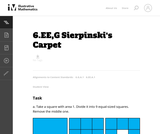Unrestricted Use
CC BY
Rating
0.0 stars

This is a task from the Illustrative Mathematics website that is one part of a complete illustration of the standard to which it is aligned. Each task has at least one solution and some commentary that addresses important asects of the task and its potential use. Here are the first few lines of the commentary for this task: Take a square with area 1. Divide it into 9 equal-sized squares. Remove the middle one. What is the area of the figure now? Take the remaining 8 square...

Subject:
Mathematics
Material Type:
Activity/Lab
Provider:
Illustrative Mathematics
Provider Set:
Illustrative Mathematics
Author:
Illustrative Mathematics
08/06/2013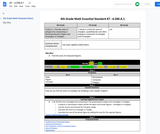Conditional Remix & Share Permitted
CC BY-NC-SA
Rating
0.0 stars

Find the area of polygons by composing or decomposing the shapes into rectangles or triangles.

Subject:
Mathematics
Material Type:
Activity/Lab
Author:
Liberty Public Schools
04/12/2021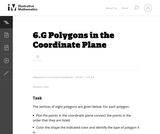Unrestricted Use
CC BY
Rating
0.0 stars

This is a task from the Illustrative Mathematics website that is one part of a complete illustration of the standard to which it is aligned. Each task has at least one solution and some commentary that addresses important asects of the task and its potential use. Here are the first few lines of the commentary for this task: The vertices of eight polygons are given below. For each polygon: * Plot the points in the coordinate plane connect the points in the order that they a...

Subject:
Mathematics
Material Type:
Activity/Lab
Provider:
Illustrative Mathematics
Provider Set:
Illustrative Mathematics
Author:
Illustrative Mathematics
09/08/2013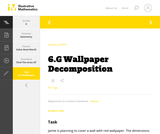Unrestricted Use
CC BY
Rating
0.0 stars

This is a task from the Illustrative Mathematics website that is one part of a complete illustration of the standard to which it is aligned. Each task has at least one solution and some commentary that addresses important aspects of the task and its potential use.

Subject:
Geometry
Material Type:
Activity/Lab
Provider:
Illustrative Mathematics
Provider Set:
Illustrative Mathematics
Author:
Illustrative Mathematics
08/06/2015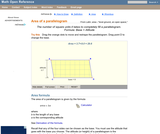Rating
0.0 stars

A web page and interactive applet showing the ways to calculate the area of a parallelogram. The user can drag the vertices of the parallelogram and the other points change automatically to ensure it remains a parallelogram. A grid inside the shape allows students to estimate the area visually, then check against the actual computed area, which is continuously recomputed and displayed. The text on the page gives three different ways to calculate the area with a formula for each. The applet uses one of the methods to compute the area in real time, so it changes as the rhombus is reshaped with the mouse. A companion page is http://www.mathopenref.com/parallelogram.html showing the definition and properties of a parallelogram Applet can be enlarged to full screen size for use with a classroom projector. This resource is a component of the Math Open Reference Interactive Geometry textbook project at http://www.mathopenref.com.

Subject:
Geometry
Material Type:
Simulation
Provider:
Math Open Reference
Author:
John Page
02/16/2011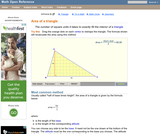Rating
0.0 stars

An interactive applet and associated web page that explain the area of a triangle. The applet shows a triangle that can be reshaped by dragging any vertex. As it changes, the area is continually recalculated using the 'half base times height' method. The triangle has a fixed square grid in its interior that can be used to visually estimate the area for later correlation with the calculated value. The calculation can be hidden while estimation is in progress. The text page has links to a similar page that uses Heron's Formula to compute the area. Applet can be enlarged to full screen size for use with a classroom projector. This resource is a component of the Math Open Reference Interactive Geometry textbook project at http://www.mathopenref.com.

Subject:
Geometry
Material Type:
Simulation
Provider:
Math Open Reference
Author:
John Page
02/16/2011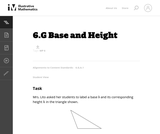Unrestricted Use
CC BY
Rating
0.0 stars

The purpose of this task is to help students understand what is meant by a base and its corresponding height in a triangle and to be able to correctly identify all three base-height pairs.

Subject:
Mathematics
Geometry
Material Type:
Activity/Lab
Provider:
Illustrative Mathematics
Provider Set:
Illustrative Mathematics
Author:
Illustrative Mathematics
05/01/2012
Remix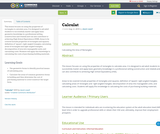Unrestricted Use
CC BY
Rating
0.0 stars

This lesson focuses on using the properties of rectangles to calculate area. It is designed to aid adult students to successfully master and apply basic geometry knowledge in a professional setting (construction and related) and can also contribute to achieving High School Equivalency (HSE).
Areas to be covered include properties of rectangles and squares; definition of ‘square’; right-angled triangles; calculating areas of rectangles and right-angled triangles; decomposition of area into manageable units; and calculating costs. Students will apply this knowledge to calculating the costs of purchasing building materials.

Subject:
Mathematics
Material Type:
Interactive
Lesson Plan
Author:
david cowell
08/08/2019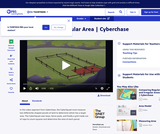Educational Use
Rating
4.0 stars

In this video segment from Cyberchase, the CyberSquad measures Judge Trudy's land claim by using tarps, fence posts and a grid made with rope.

Subject:
Mathematics
Material Type:
Lecture
Provider:
PBS LearningMedia
Provider Set:
PBS Learning Media: Multimedia Resources for the Classroom and Professional Development
Author:
U.S. Department of Education
WNET
07/07/2008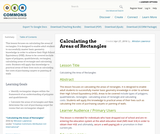Unrestricted Use
CC BY
Rating
0.0 stars

This lesson focuses on calculating the areas of rectangles. It is designed to enable adult students to successfully master basic geometry knowledge in order to achieve their High School Equivalency (HSE). Areas to be covered include types of polygons, quadrilaterals, rectangles; calculating areas of rectangle and calculating costs. Students will apply this knowledge to practical areas of their lives such as calculating the costs of purchasing carpets or painting of walls

Subject:
Mathematics
Material Type:
Interactive
Lesson Plan
Author:
Winston Lawrence
05/05/2016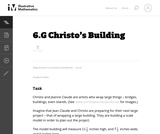Unrestricted Use
CC BY
Rating
0.0 stars

This task is primarily about volume and surface area, although it also gives students an early look at converting between measurements in scale models and the real objects they correspond to.

Subject:
Mathematics
Geometry
Material Type:
Activity/Lab
Provider:
Illustrative Mathematics
Provider Set:
Illustrative Mathematics
Author:
Illustrative Mathematics
05/01/2012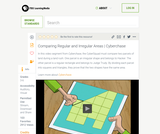Educational Use
Rating
3.0 stars

The CyberSquad proves that the area of Hacker's land is equal to the area of Judge Trudy's land in this video segment from Cyberchase.

Subject:
Mathematics
Material Type:
Lecture
Provider:
PBS LearningMedia
Provider Set:
PBS Learning Media: Multimedia Resources for the Classroom and Professional Development
Author:
U.S. Department of Education
WNET
07/08/2008Only Sharing Permitted
CC BY-NC-ND
Rating
4.0 stars

This lesson unit is intended to help teachers assess how well students are able to: Select appropriate mathematical methods to use for an unstructured problem; interpret a problem situation, identifying constraints and variables, and specify assumptions; work with 2- and 3-dimensional shapes to solve a problem involving capacity and surface area; and communicate their reasoning clearly.

Subject:
Geometry
Material Type:
Assessment
Lesson Plan
Provider:
Shell Center for Mathematical Education
Provider Set:
Mathematics Assessment Project (MAP)
04/26/2013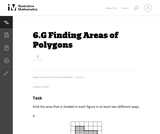Unrestricted Use
CC BY
Rating
0.0 stars

The purpose of this task is to have students work on a sequence of area problems that shows the advantage of increasingly abstract strategies in preparation for developing general area formulas for parallelograms and triangles.

Subject:
Mathematics
Geometry
Material Type:
Activity/Lab
Provider:
Illustrative Mathematics
Provider Set:
Illustrative Mathematics
Author:
Illustrative Mathematics
05/01/2012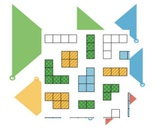Conditional Remix & Share Permitted
CC BY-NC
Rating
0.0 stars

Download GeoGebra Illustrative Mathematics LMS course files. All of GeoGebra's Illustrative Mathematics apps have been placed via links into Moodle courses - each unit is one course. There are twenty save middles school courses and more for high school (the list is growing.) These course files can then be uploaded into Moodle, Schoology, Canvas or D2L.

Subject:
Mathematics
Material Type:
Full Course
Author:
Dan MGuire
Diana Fisher Ph.D.
04/07/2021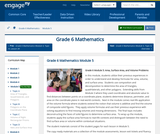Conditional Remix & Share Permitted
CC BY-NC-SA
Rating
0.0 stars

In this module, students utilize their previous experiences in order to understand and develop formulas for area, volume, and surface area.  Students use composition and decomposition to determine the area of triangles, quadrilaterals, and other polygons.  Extending skills from Module 3 where they used coordinates and absolute value to find distances between points on a coordinate plane, students determine distance, perimeter, and area on the coordinate plane in real-world contexts.  Next in the module comes real-life application of the volume formula where students extend the notion that volume is additive and find the volume of composite solid figures.  They apply volume formulas and use their previous experience with solving equations to find missing volumes and missing dimensions.  The final topic includes deconstructing the faces of solid figures to determine surface area.  To wrap up the module, students apply the surface area formula to real-life contexts and distinguish between the need to find surface area or volume within contextual situations.

Subject:
Geometry
Material Type:
Module
Provider:
New York State Education Department
Provider Set:
EngageNY
01/31/2014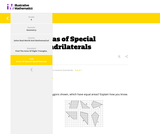Conditional Remix & Share Permitted
CC BY-NC-SA
Rating
0.0 stars

This task asks students to identify which of the six polygons have the same area. Students may complete the task using a variety of techniques including decomposing shapes, using transformations (rotations, reflections, translations) to move one or more parts of the figure to another part to more easily calculate the area, enclosing the polygon inside a larger rectangle and then subtract the areas of the "extra" pieces, etc.

Subject:
Mathematics
Material Type:
Activity/Lab
Diagram/Illustration
Lesson Plan
Provider:
Illustrative Mathematics
05/08/2020Only Sharing Permitted
CC BY-NC-ND
Rating
4.0 stars

This lesson unit is intended to help you assess how well students are able to: Perform arithmetic operations, including those involving whole-number exponents, recognizing and applying the conventional order of operations; Write and evaluate numerical expressions from diagrammatic representations and be able to identify equivalent expressions; apply the distributive and commutative properties appropriately; and use the method for finding areas of compound rectangles.

Subject:
Geometry
Material Type:
Assessment
Lesson Plan
Provider:
Shell Center for Mathematical Education
Provider Set:
Mathematics Assessment Project (MAP)
04/26/2013Conditional Remix & Share Permitted
CC BY-NC
Rating
0.0 stars

Subject:
Mathematics
Material Type:
Full Course
Provider:
Pearson